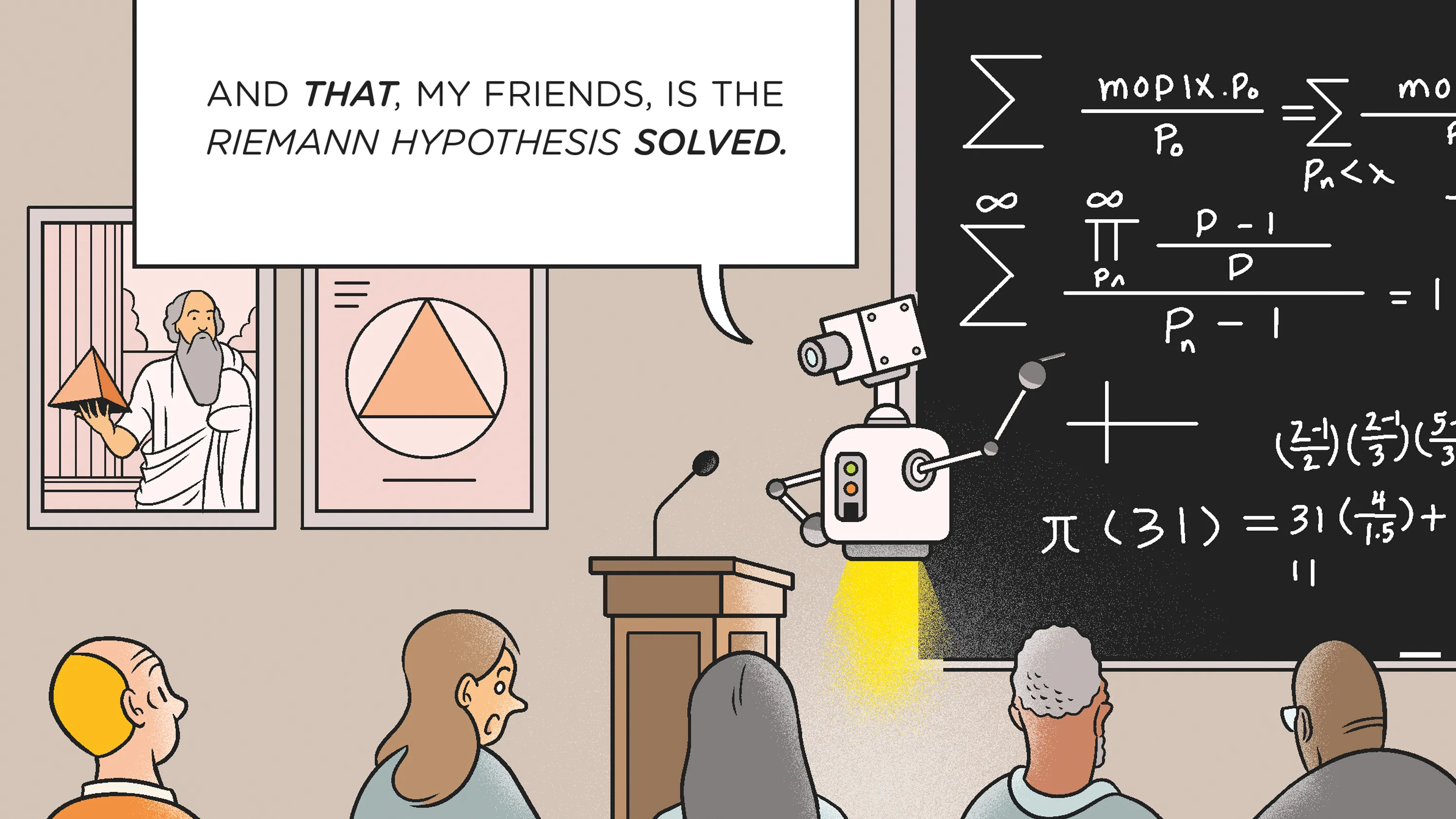top of page
Search
• community959

# A.I. Is Coming for Mathematics, TooBy: Louie Chiang

A 17th-century portrait of the ancient Greek mathematician Euclid, his dirty hands holding Elements, his famous book on mathematics, may be found at the Getty Museum in Los Angeles. For nearly two millennia, Euclid's book functioned as a model of mathematical argumentation, with the following phases building complicated equations on basic conceptions and preceding theorems.

By the twentieth century, however, mathematicians sought to base mathematics in formal systems rather than basic, Euclidean ideas about geometry. This change created a need for exact symbolic representations and mechanical laws, allowing mathematics to be translated into computer code.

The four-color theorem, which states that four colors are sufficient to color any map so that no two adjacent regions have the same color, was proved using brute computer force in 1976. It is an important theorem in graph theory and had been a longstanding problem in mathematics.

Today, mathematicians must deal with another transformational force: artificial intelligence. Christian Szegedy, a former Google computer scientist, predicts that computer systems will match or exceed the problem-solving ability of human mathematicians within a decade.

Workshops and meetings bring together mathematicians and computer scientists to investigate "machine-assisted proofs" and the consequences of the integration of AI into mathematics. Mathematicians such as Akshay Venkatesh, of the Institute for Advanced Study in Princeton, support the conscious use of technology to grow human knowledge while recognizing the need for caution.

Mathematicians now rely on computerized reasoning tools to create proofs. Despite certain difficulties, these methods provide line-by-line feedback and improve instructional effectiveness.

As demonstrated by Google's DeepMind, machine learning enhances mathematics by directing human senses. Applications like AlphaFold for protein folding highlight the ability of AI to handle challenges beyond the capability of humans.

As mathematicians explore this new territory, they must strike a balance between logic and intuition. These mathematicians look forward to a future where artificial intelligence enhances human intelligence, enabling the resolution of complex problems.

Mathematicians are combining the transformational promise of technology with the timeless elegance of mathematical study by carefully incorporating AI into their field. This investigation has the potential to push the limits of knowledge and usher in a new age of mathematical discovery.